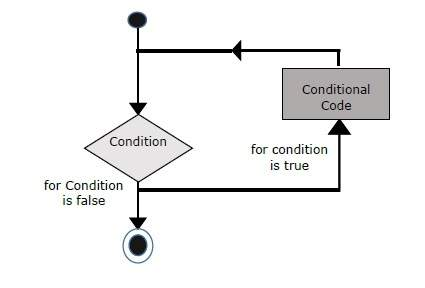# JavaScript - For Loop

The 'for' loop is the most compact form of looping. It includes the following three important parts −
• The loop initialization where we initialize our counter to a starting value. The initialization statement is executed before the loop begins.
• The test statement which will test if a given condition is true or not. If the condition is true, then the code given inside the loop will be executed, otherwise the control will come out of the loop.
• The iteration statement where you can increase or decrease your counter.
You can put all the three parts in a single line separated by semicolons.

## Flow Chart

The flow chart of a for loop in JavaScript would be as follows −### Syntax

The syntax of for loop is JavaScript is as follows −
```for (initialization; test condition; iteration statement){
Statement(s) to be executed if test condition is true
}```

### Example

Try the following example to learn how a for loop works in JavaScript.
```<html>
<body>

<script type="text/javascript">
<!--
var count;
document.write("Starting Loop" + "<br />");

for(count = 0; count < 10; count++){
document.write("Current Count : " + count );
document.write("<br />");
}

document.write("Loop stopped!");
//-->
</script>

<p>Set the variable to different value and then try...</p>
</body>
</html>```

### Output

```Starting Loop
Current Count : 0
Current Count : 1
Current Count : 2
Current Count : 3
Current Count : 4
Current Count : 5
Current Count : 6
Current Count : 7
Current Count : 8
Current Count : 9
Loop stopped!
Set the variable to different value and then try...```